You’ve reached the end of your free Videos limit.
#5 | Field due to Circle, Semi Circle & Arc
(Physics) > Moving Charges and Magnetism
Unable to watch the video, please try another server
Related Practice Questions :

Two similar coils of radius R are lying concentrically with their planes at right angles to each other. The currents flowing in them are 3I and 4I respectively. The resultant magnetic field induction at the center is :

1.

2.

3.

4.   $\mathrm{Zero}$

High Yielding Test Series + Question Bank - NEET 2020

Difficulty Level:

A current wire is hidden in a wall. Its position can be located with the help of a

1.  Moving coil galvanometer

2.  Voltmeter

3.  Hotwire ammeter

4.  Magnetic needle

High Yielding Test Series + Question Bank - NEET 2020

Difficulty Level:

Which of the following graph correctly represents the variation of magnetic field induction with distance due to a thin wire carrying current?

1.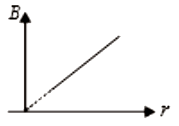2.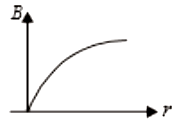3.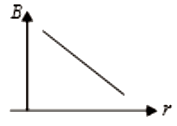4.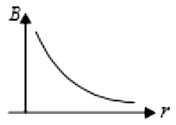High Yielding Test Series + Question Bank - NEET 2020

Difficulty Level:

1.  R

2.  2R

3.  3R

4.  $\frac{\mathrm{R}}{2}$

High Yielding Test Series + Question Bank - NEET 2020

Difficulty Level:

The magnetic dipole moment of the given loop is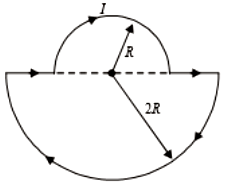1.

2.

3.

4.  $5{\mathrm{\pi R}}^{2}\mathrm{I}$